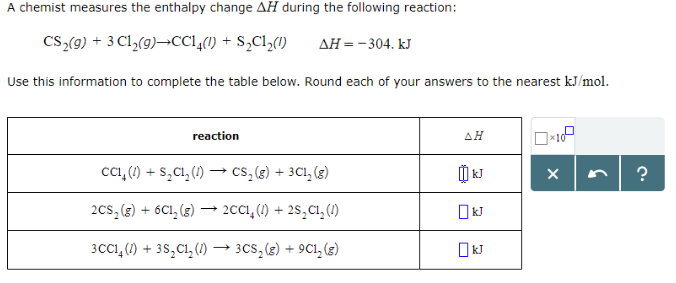Chemistry Practice Problems Hess's Law Practice Problems Solution: A chemist measures the enthalpy change ΔH during t...

🤓 Based on our data, we think this question is relevant for Professor Hinton's class at UTOLEDO.

# Solution: A chemist measures the enthalpy change ΔH during the following reaction: CS2 (g) + 3Cl2 (g) → CCl4 (l) + S2Cl2 (l)     ΔH = -304. kJ Use this information to complete the table below. Round each of your answers to the nearest kJ/mol.

###### Problem

A chemist measures the enthalpy change ΔH during the following reaction:

CS2 (g) + 3Cl2 (g) → CCl4 (l) + S2Cl2 (l)     ΔH = -304. kJ

Use this information to complete the table below. Round each of your answers to the nearest kJ/mol.View Complete Written Solution

Hess's Law

Hess's Law

#### Q. A chemist measures the enthalpy change ΔH during the following reaction: P4 (s) + 6Cl2 (g) → 4 PCl3 (g)       ΔH = -1148.kJ Use this information to co...

Solved • Fri Sep 14 2018 12:37:47 GMT-0400 (EDT)

Hess's Law

#### Q. A chemist measures the enthalpy change ΔH during the following reaction: 2H2O (l) + O2 (g) → 2H2O2 (l)            ΔH = 196. kJ Use this information to...

Solved • Fri Sep 14 2018 12:23:18 GMT-0400 (EDT)

Hess's Law

#### Q. Given the following data (1) 2ClF (g) + O2 (g) → Cl2O (g) + OF2 (g)         ΔH°rxn = 167.5 kJ (2) 2F2 (g) + O2 (g) → 2OF2 (g)                         ...

Solved • Tue Aug 21 2018 14:36:51 GMT-0400 (EDT)

Hess's Law

#### Q. Use the following data to calculate the standard heat (enthalpy) of formation, ΔH °f, of manganese(IV) oxide, MnO2 (s). 2MnO2 (s) → 2MnO (s) + O2 (g) ...

Solved • Tue Aug 21 2018 14:24:03 GMT-0400 (EDT)